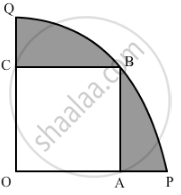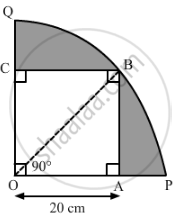# a square OABC is inscribed in a quadrant OPBQ of a circle. If OA = 20 cm, find the area of the shaded region. - Mathematics

In fig. 3, a square OABC is inscribed in a quadrant OPBQ of a circle. If OA = 20 cm, find the area of the shaded region. (Use π = 3.14)#### Solution

Let us join OB.In ΔOAB:
OB2 = OA2 + AB2  = (20)2 + (20)2 = 2 ×  (20)2

⇒ OB = 20 √2

Radius of the circle, r = 20 sqrt2 cm

"Area of qudrant OBPQ"=90^@/360^@xx3.14xx(20sqrt2)^2

=90/360xx3.14xx(20sqrt2)^2 cm^2

=1/4xx3.14xx800 cm^2

=628 cm^2

Area of square OABC = (Side)2 = (20)2 cm2 = 400 cm2

∴ Area of the shaded region = Area of quadrant OPBQ − Area of square OA

= (628 − 400) cm2

= 228 cm2

Is there an error in this question or solution?
Chapter 12: Areas Related to Circles - Exercise 12.3 [Page 237]

#### APPEARS IN

NCERT Class 10 Maths
Chapter 12 Areas Related to Circles
Exercise 12.3 | Q 13 | Page 237
Share